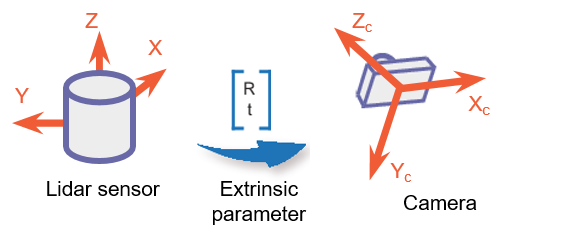## What Is Lidar Camera Calibration?

For applications such as automated driving, robotics, navigation systems, and 3-D scene reconstruction, data of the same scene is often captured using both lidar and camera sensors. To accurately interpret the objects in a scene, it is necessary to fuse the lidar and the camera outputs together. Lidar camera calibration estimates a rigid transformation matrix that establishes the correspondences between the points in the 3-D lidar plane and the pixels in the image plane. There are two parts to lidar camera calibration:

• Calibration for intrinsic parameters

• Calibration for extrinsic parameters between the lidar and camera

The intrinsic parameters of the lidar sensors are calibrated in advance by the manufacturers.### Extrinsic Calibration of Lidar and Camera

Extrinsic calibration of lidar and camera sensors generally uses calibration objects, such as planar boards with chessboard patterns, in the captured scene. The corner points of the calibration object are detected in the data captured by each sensor and used to establish the point correspondences between them. You can compute the image plane coordinates corresponding to the 3-D lidar points by using the extrinsic calibration and the intrinsic camera parameters.The extrinsic calibration is a rigid transformation that maps points from the 3-D lidar coordinate system to the 3-D camera coordinate system. The extrinsic parameters consist of a rotation, R, and a translation, t.You can estimate the rigid transformation matrix by using the `estimateLidarCameraTransform` function.

Then, compute the 2-D image plane coordinates from the 3-D lidar points and the extrinsic parameter.K is the camera intrinsic matrix defined by the intrinsic parameters: focal length, optical center (also known as the principal point), and skew coefficient.

`$K=\text{\hspace{0.17em}}\text{\hspace{0.17em}}\left[\begin{array}{ccc}{f}_{x}& 0& 0\\ s& {f}_{y}& 0\\ {c}_{x}& {c}_{y}& 1\end{array}\right]$`

 $\left[\begin{array}{cc}{c}_{x}& {c}_{y}\end{array}\right]$ — Optical center (the principal point), in pixels. $\left(\begin{array}{cc}{f}_{x},& {f}_{y}\end{array}\right)$ — Focal length in pixels. ${f}_{x}=F/{p}_{x}$ ${f}_{y}=F/{p}_{y}$ F — Focal length in world units, typically expressed in millimeters. $\left(\begin{array}{cc}{p}_{x},& {p}_{y}\end{array}\right)$ — Size of the pixel in world units. $s$ — Skew coefficient, which is non-zero if the image axes are not perpendicular. $s={f}_{x}\mathrm{tan}\alpha$
The pixel skew is defined as:You can estimate the camera intrinsic parameters by using the `cameraIntrinsics` function. Using the estimated extrinsic calibration and camera intrinsic parameters, you can project lidar points onto the image or fuse the camera and the lidar sensor outputs. For more details, see the `projectLidarPointsOnImage` and `fuseCameraToLidar` functions.

 Zhou, Lipu, Zimo Li, and Michael Kaess. “Automatic Extrinsic Calibration of a Camera and a 3D LiDAR Using Line and Plane Correspondences.” In 2018 IEEE/RSJ International Conference on Intelligent Robots and Systems (IROS), 5562–69. Madrid: IEEE, 2018. https://doi.org/10.1109/IROS.2018.8593660.

## SupportGet trial now